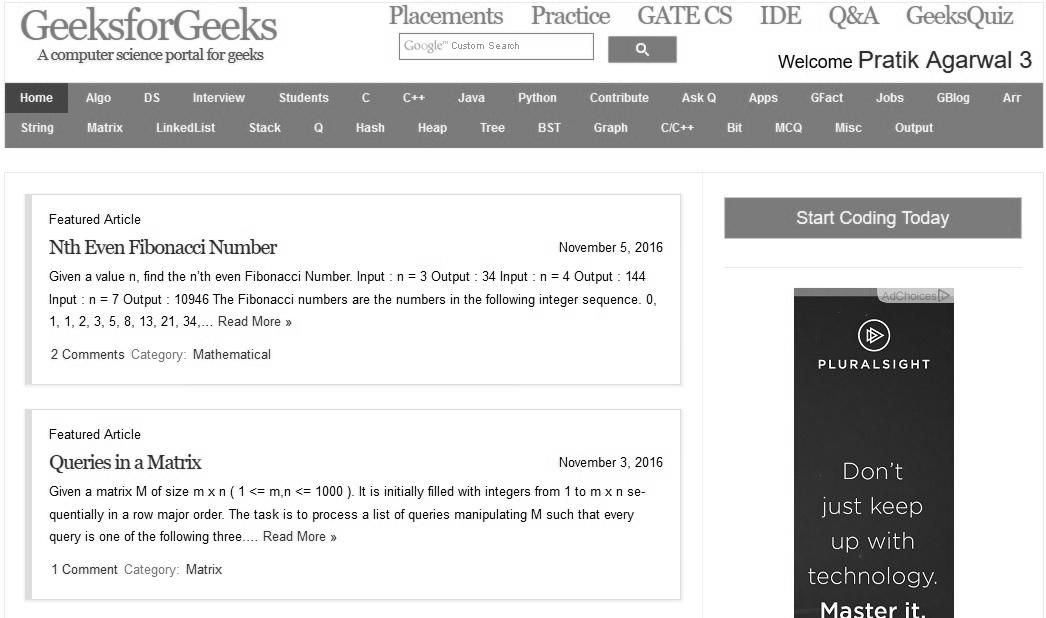Related Articles
Image Processing in Java | Set 12 ( Contrast Enhancement )
• Difficulty Level : Hard
• Last Updated : 16 Jun, 2017

In this article we will learn how to enhance contrast of an image using OpenCV library. In order to enhance contrast histogram equalization techniques are used.

At first we need to setup OpenCV for Java, we recommend to use eclipse for the same since it is easy to use and setup. For installation refer to http://docs.opencv.org/2.4/doc/tutorials/introduction/java_eclipse/java_eclipse.html

Now lets understand some of the methods required for contrast enhancement.

1. equalizeHist(source, destination) – This method resides in Imgproc package of OpenCv. source parameter is 8-bit single channel source image, and the destination parameter is the destination image
2. Imcodecs.imread()/Imcodecs.imwrite() – These methods are used to read and write images as Mat objects which are rendered by OpenCV.

 `// Java program to demonstrate contrast enhancement ` `package` `ocv; ` `import` `org.opencv.core.Core; ` `import` `org.opencv.core.Mat; ` `import` `org.opencv.imgcodecs.Imgcodecs; ` `import` `org.opencv.imgproc.Imgproc; ` ` `  `public` `class` `Main ` `{ ` `    ``public` `static` `void` `main( String[] args ) ` `    ``{ ` `        ``try` `        ``{ ` `            ``// For proper execution of native libraries ` `            ``// Core.NATIVE_LIBRARY_NAME must be loaded before ` `            ``// calling any of the opencv methods ` `            ``System.loadLibrary( Core.NATIVE_LIBRARY_NAME ); ` ` `  `            ``//input image ` `            ``Mat source = Imgcodecs.imread(``"E:\\input.jpg"``, ` `                           ``Imgcodecs.CV_LOAD_IMAGE_GRAYSCALE); ` `            ``Mat destination = ``new` `Mat(source.rows(), ` `                               ``source.cols(), source.type()); ` ` `  `            ``// applying histogram equalization ` `            ``Imgproc.equalizeHist(source, destination); ` ` `  `            ``// writing output image ` `            ``Imgcodecs.imwrite(``"E:\\output.jpg"``, destination); ` `        ``} ` `        ``catch` `(Exception e) ` `        ``{ ` `            ``System.out.println(``"error: "` `+ e.getMessage()); ` `        ``} ` `    ``} ` `} `

Note: The code will not work in online ide since it requires image in hard drive.

Input.jpgOutput.jpgThis article is contributed by Pratik Agarwal. If you like GeeksforGeeks and would like to contribute, you can also write an article using contribute.geeksforgeeks.org or mail your article to contribute@geeksforgeeks.org. See your article appearing on the GeeksforGeeks main page and help other Geeks.